Smartick is an advanced online program that teaches kids math and coding in only 15 min. a day

Aug20

# Practicing with Units of Measurement in the Metric System

In today’s post, we are going to take a look at activities that you can practice in your everyday life, as a game, to familiarize yourself with units of measurement in the Metric System.

With the activities’ help, you’ll stop seeing units of measurement as “abstract entities,” with which you perform calculations guided by rules that you may not end up understanding.

If you would like to review some units of measurement, you can do so by clicking here.

### Activities for Practicing with Units of Measurement: Mass

You can practice these units of measurement by using something as simple as a scale and a pack of beans.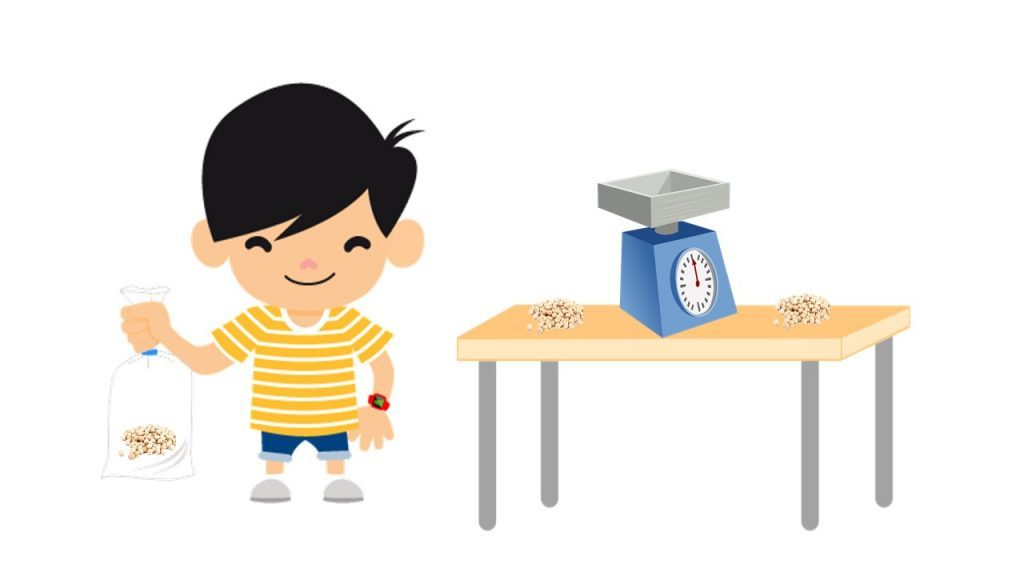You can fill small bags with specific quantities of beans, to practice using the scale and conversions between different units of measurement.

Grab a 1 kg pack of beans.

You will have exactly 1,000 g of beans, as one kilogram is one thousand grams (kilo comes from Greek and means thousand).

• With this quantity, you can make bags of 100 g of beans, and each bag’s mass would equal 1 hg (hectogram; hecto indicates a factor of one hundred).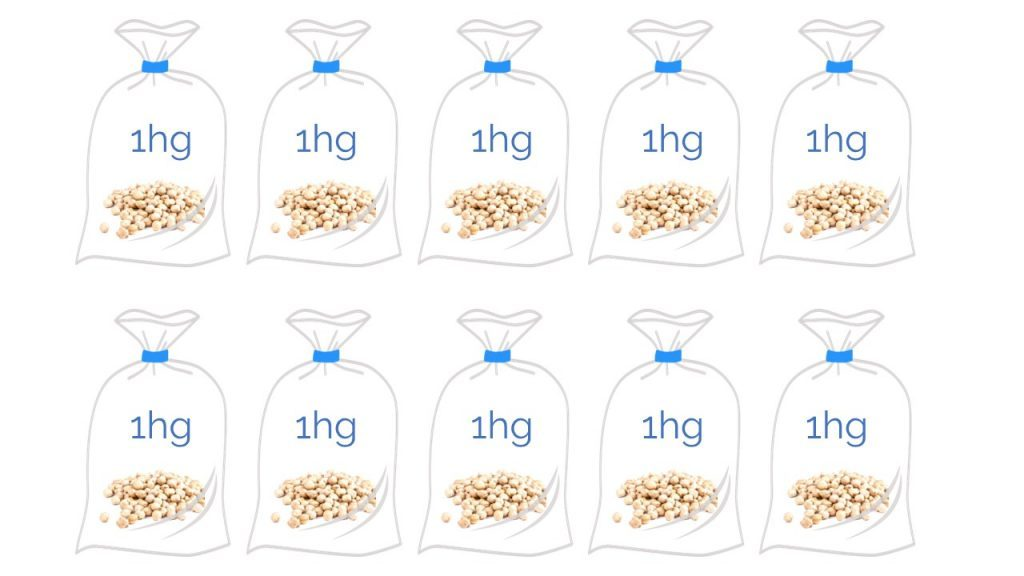Do you know how many bags of beans you will be able to make? Exactly 10!

Therefore, 10 times 1 hg equals 1 kg. It can be mathematically expressed like this:• With that pack of beans, you can also make bags weighing 10 g, and each bag would equal 1 dag (dekagram; deka/deca indicates a factor of ten).

Do you think that you would be able to make all of the possible bags? It would take you quite a while since there would be exactly 100!

So, 100 times 1 dag equals 1 kg. This is written mathematically as:### Activities for Practicing with Units of Measurement: Capacity

To practice with these units, I am sure that you have thought about using something you have around the house, that maybe you have used for a recipe before.

Let’s see, put on your thinking cap…

…a measuring cup!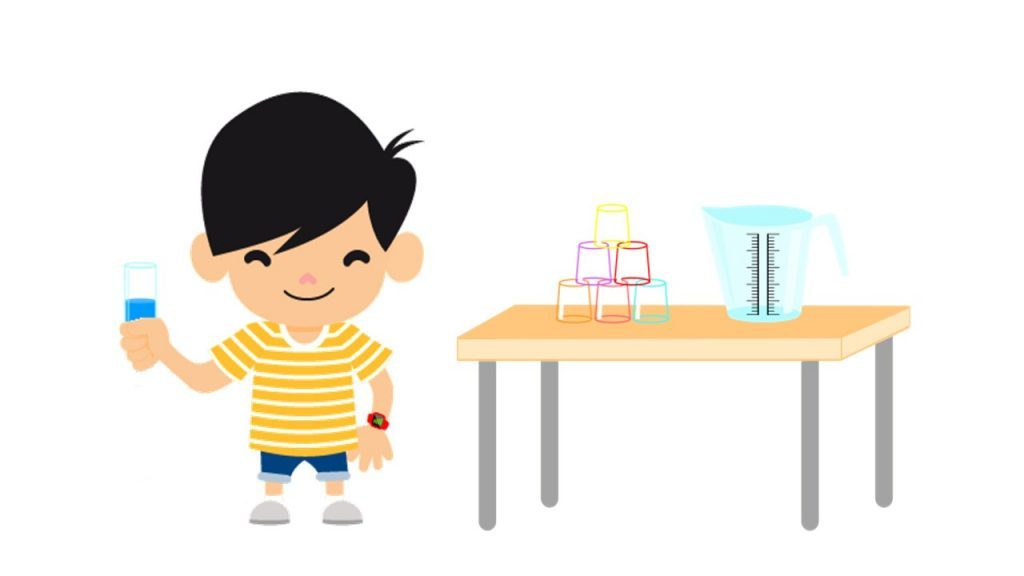You can measure how much water fits into a cup, and compare 2 different cups’ capacities, such as a taller and thinner cup versus a shorter and wider cup…the possibilities are endless!

Get a measuring cup and fill it with 1 l of water, which is 1,000 ml:

• You can distribute quantities of 100 ml of the content of the measuring cup into each cup (which is the same as 1 deciliter (dl)).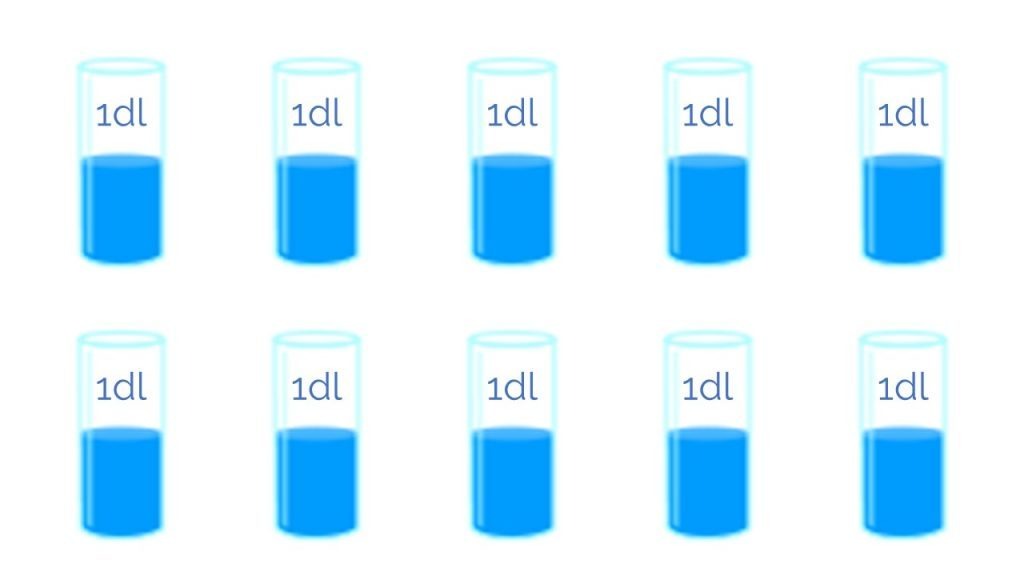Do you know how many cups we will be able to distribute the water into? 10 cups, of course!

Therefore, 10 times 1 dl equals 1 l. In math we write it out this way: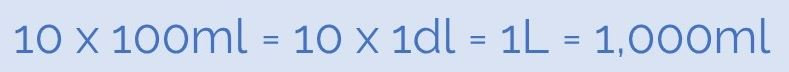• If now, with that liter of water you distribute quantities of 10 ml (which is the same as .1 cl (centiliter)) into each cup:

Do you know how many cups you could distribute the water between? Remember that 1 l =  1,000 ml!

So, it is easy to see that you could distribute it between 100 cups, right?

Therefore, 100 times 1 cl equals 1 l. Meaning: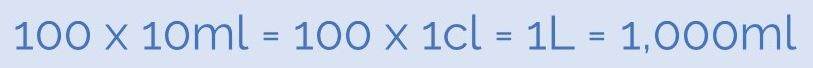### Activities for Practicing Units of Measurement: Length, Area, and Volume

You can practice these units of measurement using base ten blocks! But that is a bit of a long story, so we will explain it in another post.

Have you seen how easy it is to make unit conversions when we practice with physical objects?

I hope that you liked this post! If you did, don’t forget to share it so that others can also practice these activities.

At Smartick we have a ton of exercises for practicing with units of measurement. Register for Smartick and try it for free!